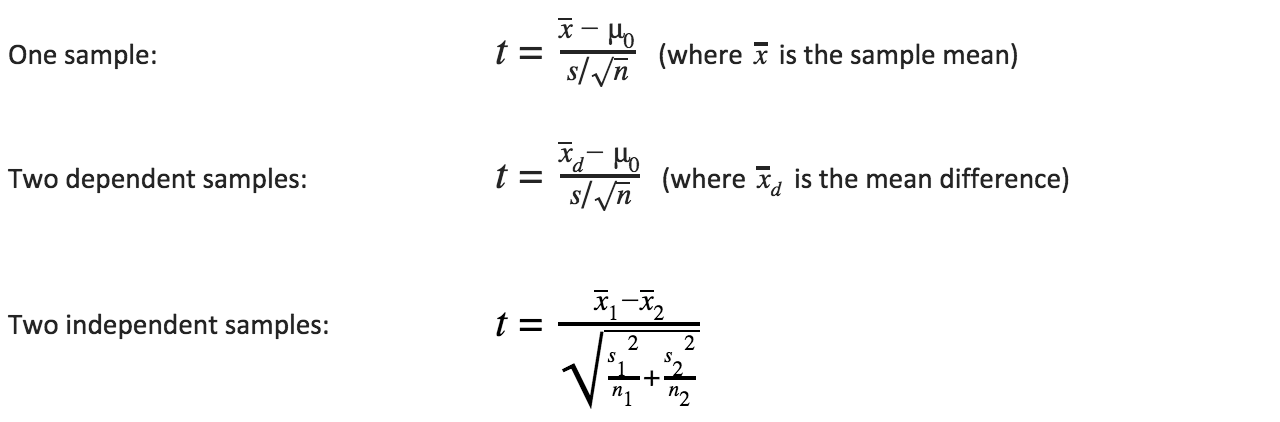PURCHASE A DIGITAL COPY PURCHASE A HARD COPY Lesson 1 Introduction to Statistical Research Methods Lesson 2 Visualizing Data Lesson 3 Central Tendency Lesson 4 Variability Lesson 5 Standardizing Lesson 6 Normal Distribution Lesson 7 Sampling Distributions Lesson 8 Estimation Lesson 9 Hypothesis Testing Lesson 10 t-Tests for Dependent Samples Lesson 11 t-Tests for Independent Samples Lesson 12 Intro to One-Way ANOVA Lesson 13 One-Way ANOVA: Test significance of differences Lesson 14 Correlation Lesson 15 Linear Regression Lesson 16 Chi-Squared Tests Afterward Index

t-Tests only test for a significant difference between two samples. If we have three samples (A, B, and C), we would have to do 3 different t-tests (AB, AC, BC); for four samples (A, B, C, and D) we would have to do 6 t-tests (AB, AC, AD, BC, BC, CD); for five samples 10 t-tests; etc.

ANOVA, which stands for “analysis of variance”, allows us to do one test for more than two samples, and tells us if at least two samples are significantly different. One-way ANOVA is used when we only have one variable, or factor.

Henceforth, we’ll denote the number of samples as k. So we have k samples, with n1 values in the first sample, n2 values in the second sample, and so on until we have nk values in the kth sample. The null and alternative hypotheses for ANOVA are:: At least two populations are significantly different

Recall that the t-statistic tells us whether or not two populations are most likely significantly different (based on the collected samples), and is a function of how far apart samples are from each other (the numerator, which is the difference between means), and the standard error (the denominator). Remember, the standard error is the estimated standard deviation of our expected population distribution (where this population is either based on a sample or the difference between dependent samples, or the result of subtracting two estimated populations based on two independent samples).When we compare three or more samples, we want the same thing: some kind of variability between means (between-group variability) divided by
a standard error (within-group variability). We’ll call this the F-statistic.

To continue learning, purchase Street-Smart Stats: A Friendly Introduction to Statistical Research Methods.